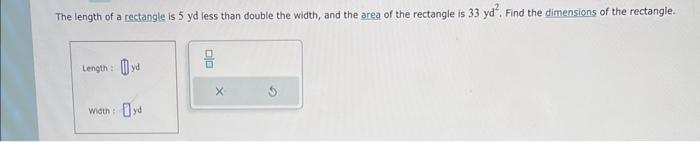Home / Expert Answers / Algebra / help-the-length-of-a-rectangle-is-5-yd-less-than-double-the-width-and-the-area-of-the-rectangl-pa358

# (Solved): help!!!!! The length of a rectangle is 5 yd less than double the width, and the area of the rectangl ...

help!!!!!The length of a rectangle is 5 yd less than double the width, and the area of the rectangle is $$33 \mathrm{yd}^{2}$$. Find the dimensions of the rectangle. \begin{tabular}{l} Length: \\ weth: Dyd \\ \hline \end{tabular}

We have an Answer from Expert